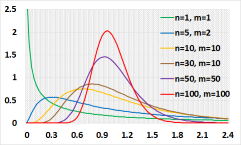﻿F Distribution

# F Distribution Calculator (Fisher-Snedecor)

Calculate F distribution or inverse F distributionexample digits=2: (0.001234 => 0.0012)
numerator degrees of freedom
denominator degrees of freedom
Choose X or P(x≤X)Message

F (Fisher Snedecor) distribution is used for a normally distributed population.

Let Z1, Z2,....Zn be independent standard random variables.
Let X1 = [Z12 + Z22 +....+ Zn2].

Let Z'1, Z'2,....Z'n be independent standard random variables.
Let X2 = [Z'12 + Z'22 +....+ Z'm2].

Let X = (X1/n) / (x2/m).

X distribute as F random variable with n degrees of freedom (numerator) and m degrees of freedom (denominator)

X1 distribute as a chi-square random variable with n degrees of freedom.
X2 distribute as a chi-square random variable with m degrees of freedom.

Example of use: ANOVA test, F test for variances comparison.